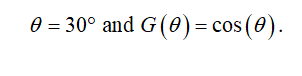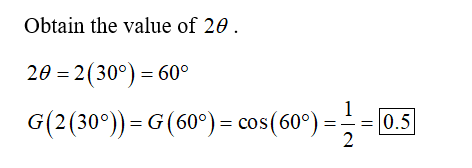# Find exact value if theta equals 30°G(theta)=cos(theta) find (2theta)G(theta)=cos(theta) find 3g(theta)

Question
17 views

Find exact value if theta equals 30°

G(theta)=cos(theta) find (2theta)

G(theta)=cos(theta) find 3g(theta)

check_circle

Given that,Step 2...

### Want to see the full answer?

See Solution

#### Want to see this answer and more?

Solutions are written by subject experts who are available 24/7. Questions are typically answered within 1 hour.*

See Solution
*Response times may vary by subject and question.
Tagged in